Module 6 - Continuity Introduction | Lesson 1 | Lesson 2 | Lesson 3 | Self-Test Lesson 6.3: Piecewise Functions In this lesson you will modify a piecewise function to make it continuous and then define the function using the with( command. Suppose the function f(x) is defined by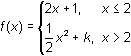6.3.1 By using the definition of continuity, find the value of k that makes the function continuous at x = 2. Click here for the answer. Graphing a Piecewise Function Display the graph of y = f(x) using the value of k that makes the function continuous. Be sure xres = 1. Return to the Home Screen and select New Problem by pressing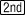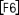Store the value found in Question 6.3.1 in k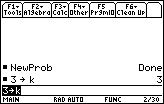In the Y= Editor, define y1 as the piecewise functionSet y1=when(x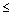2,2x+1,1/2*x^2+k) Enter thesymbol by pressing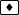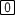.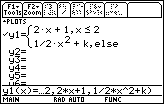Graph the function in a [-3, 7] x [-3, 25] window with xscl = 1 and yscl = 5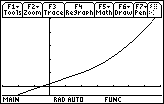The graph appears to be continuous at x = 2. 6.3.2 How would the graph change if you changed k to 7? Click here for the answer. Visualize the result of changing the value of k to 7. Change the value of k to 7 on the Home Screen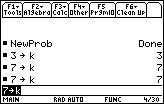Change the graphing style of y1 from Line to Dot so that the calculator will not connect the two pieces. Return to the Y= Editor and highlight y1 by using the cursor movement keys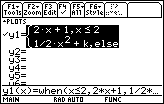While y1 is highlighted, display the Style menu by pressing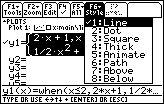Select "2:Dot" Display the graph of the function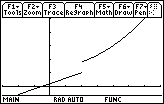The parabolic part has been shifted upward, and the function is no longer continuous at x = 2. The discontinuity in this modified function (k = 7) is a jump discontinuity. 6.3.3 Evaluate the left- and right-hand limits of the piecewise function with k = 7. Click here for the answer. < Back | Next > ©Copyright 2007 All rights reserved. | Trademarks | Privacy Policy | Link Policy